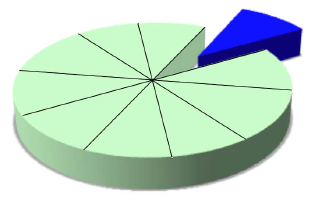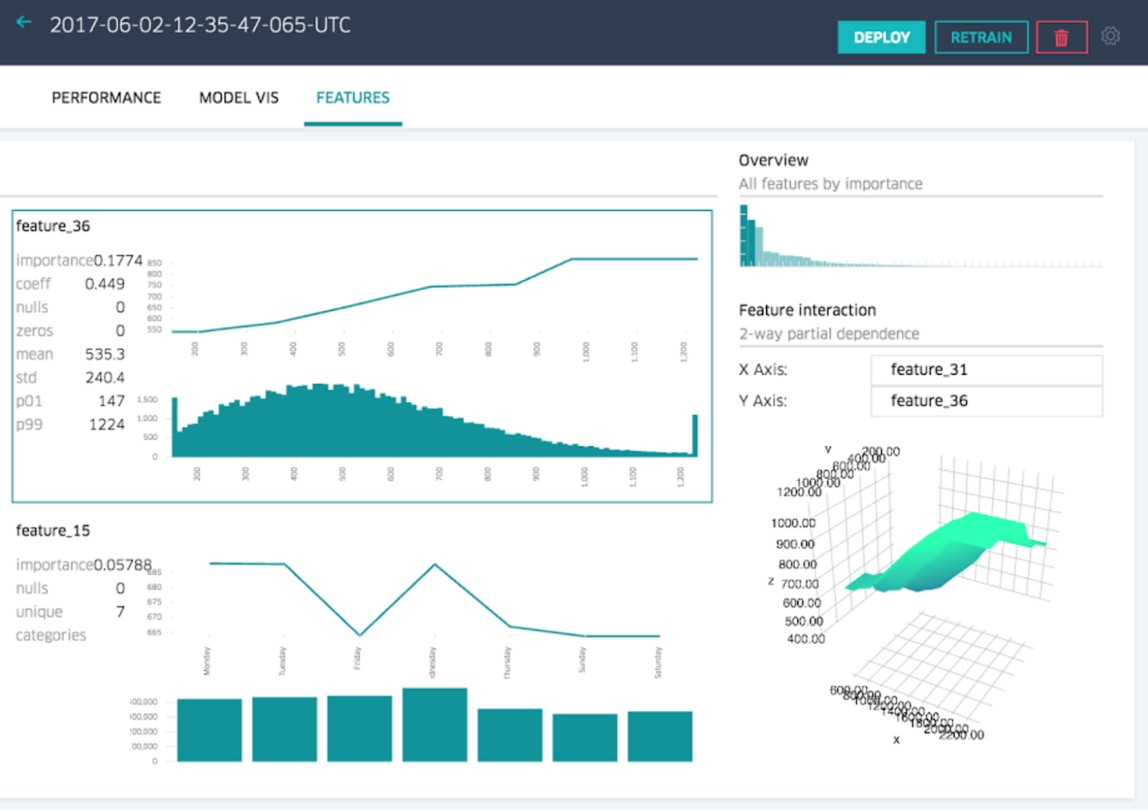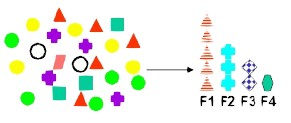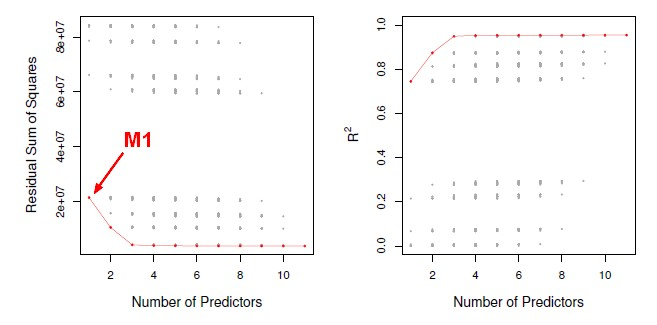# Data Mining - (Dimension|Feature) (Reduction)

In machine learning and statistics, dimensionality reduction is the process of reducing the number of random variables (features) under consideration and can be divided into:

This methods are some called “Model selection methods”.

They are an essential tool for data analysis, especially for big datasets involving many predictors.

In dimensionality reduction, the goal is to select/retain a subset of features while still retaining as much of the variance in the dataset as possible.

## Concept

• random projections
• feature hashing

## Documentation / Reference

Discover More(Statistics|Data Mining) - (K-Fold) Cross-validation (rotation estimation)

Cross-validation, sometimes called rotation estimation is a resampling validation technique for assessing how the results of a statistical analysis will generalize to an independent new data set. This...Data Mining - (Attribute|Feature) (Selection|Importance)

Feature selection is the second class of dimension reduction methods. They are used to reduce the number of predictors used by a model by selecting the best d predictors among the original p predictors....Data Mining - (Feature|Attribute) Extraction Function

Feature extraction is the second class of methods for dimension reduction. dimension reduction It creates new attributes (features) using linear combinations of the (original|existing) attributes. ...Data Mining - Feature Hashing

With a feature hashing function, the number of bucket becomes the number of features leading to a dimension reduction Spark Data...Machine Learning - (Overfitting|Overtraining|Robust|Generalization) (Underfitting)

A learning algorithm is said to overfit if it is: more accurate in fitting known data (ie training data) (hindsight) but less accurate in predicting new data (ie test data) (foresight) Ie the model...Statistics - Factor Analysis

Factor analysis is a feature extraction statistical method used to describe variability among observed, correlated variables in terms of a potentially lower number of unobserved variables called factors....Statistics - Model Selection

Model selection is the task of selecting a statistical model from a set of candidate models through the use of criteria's Dimension reduction procedures generates and returns a sequence of possible...